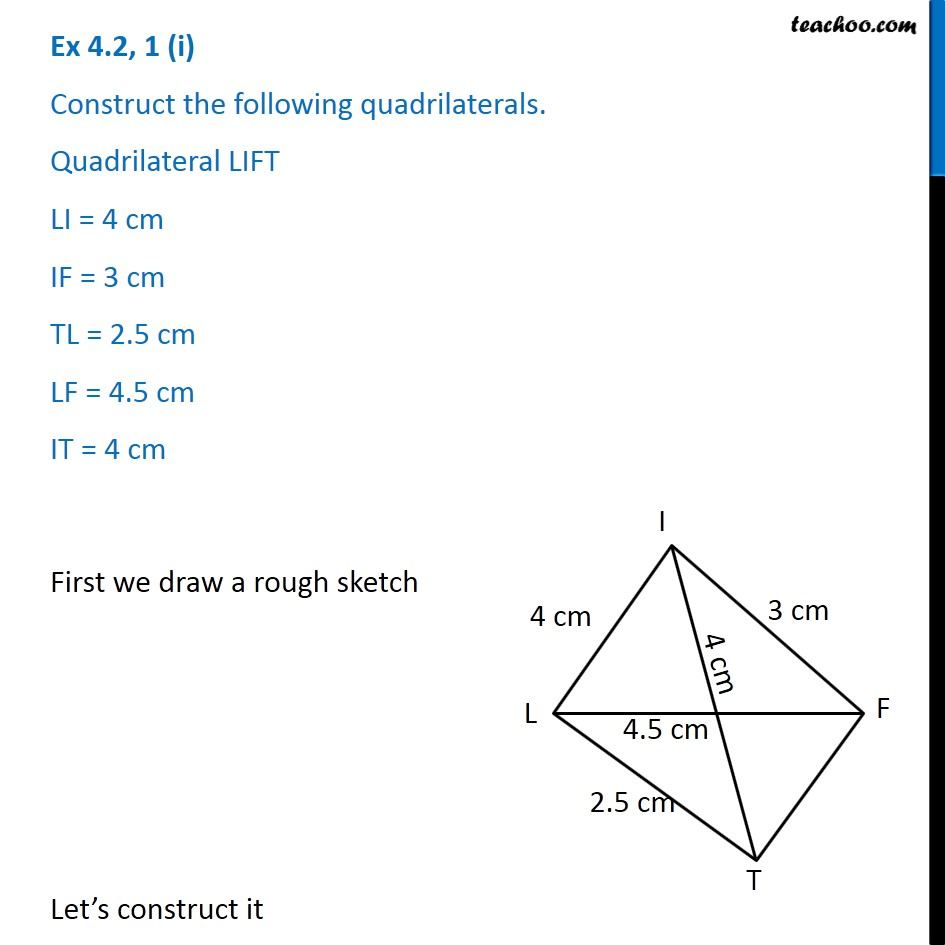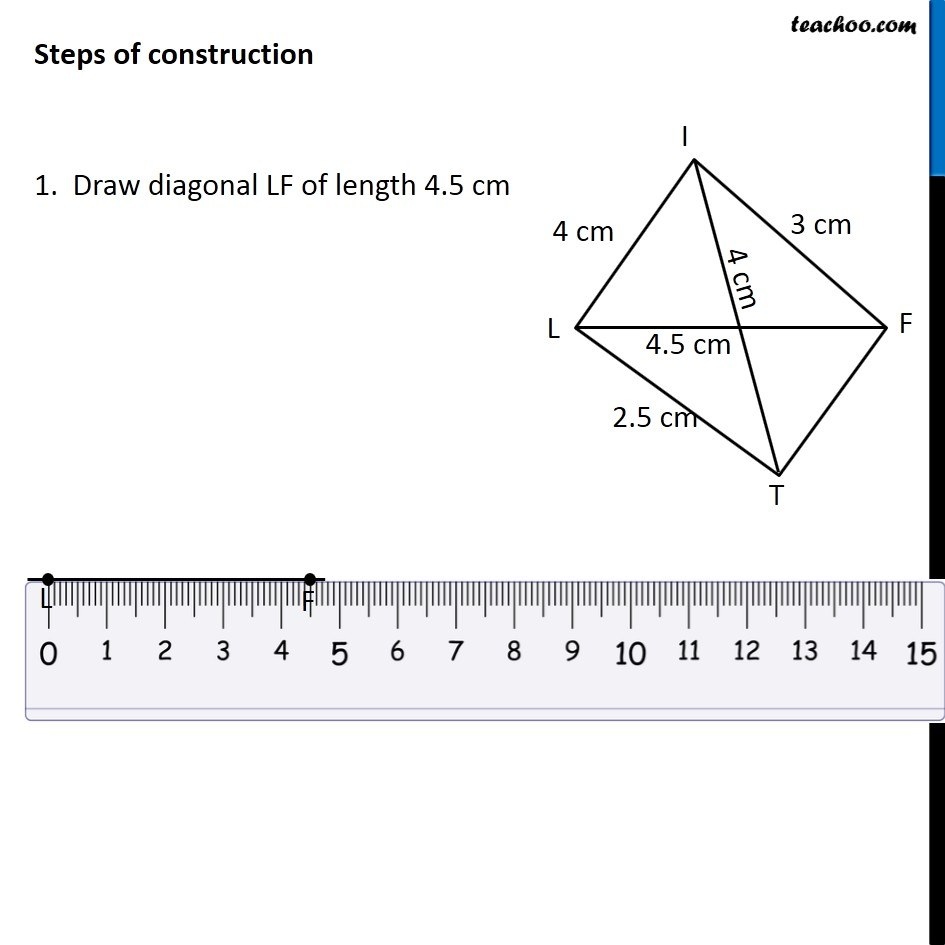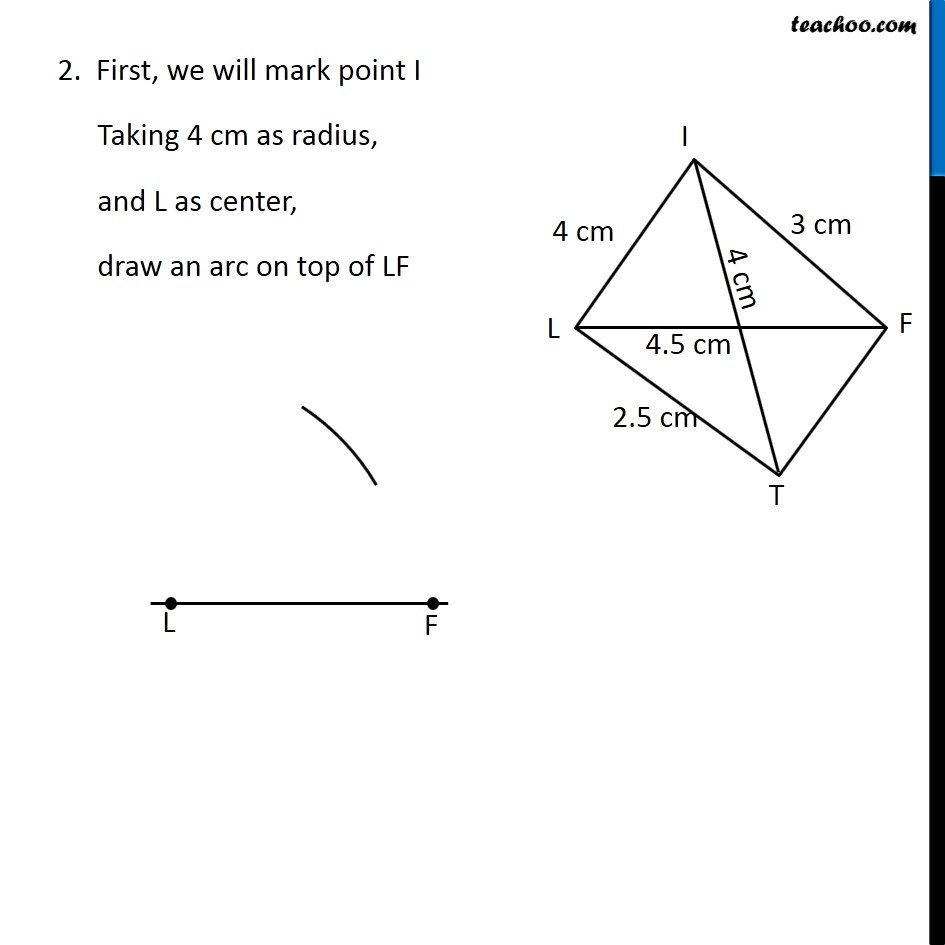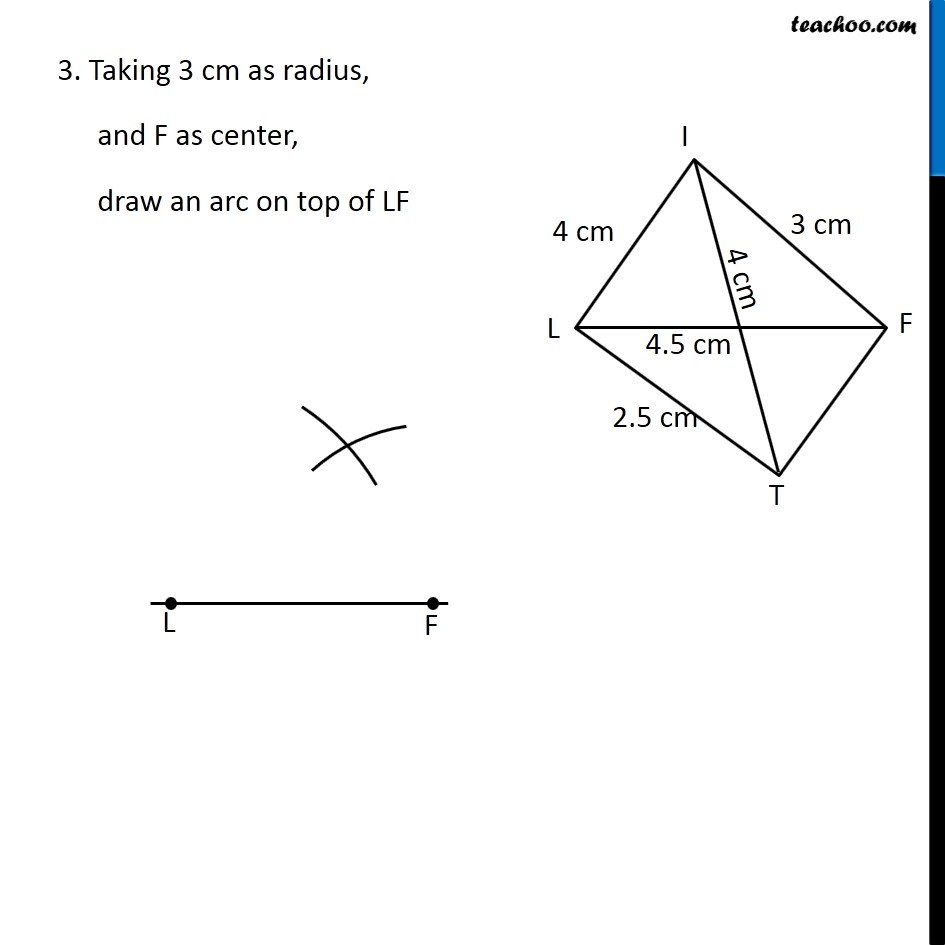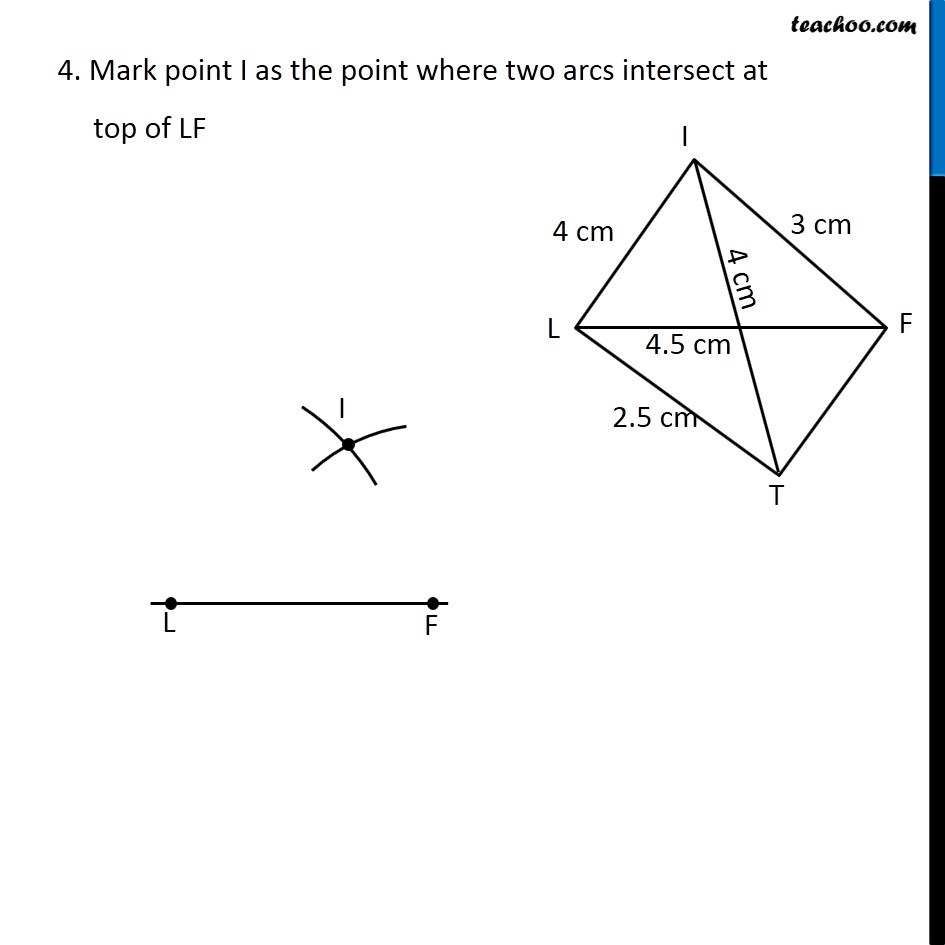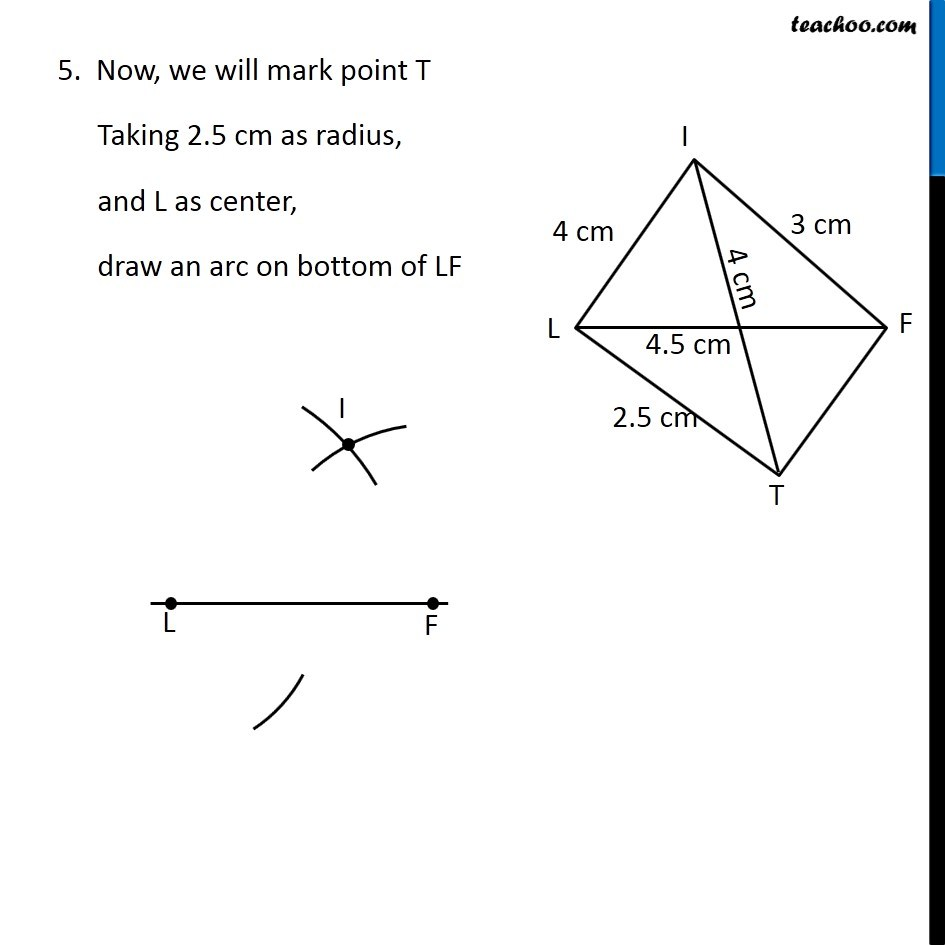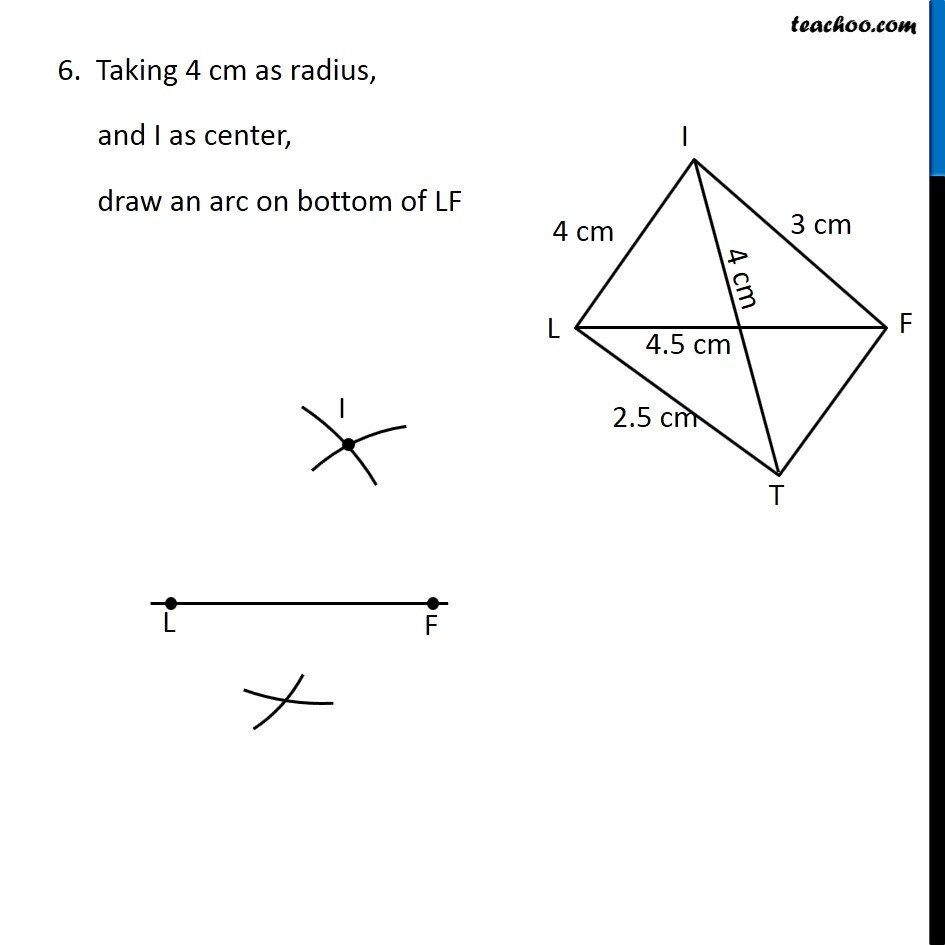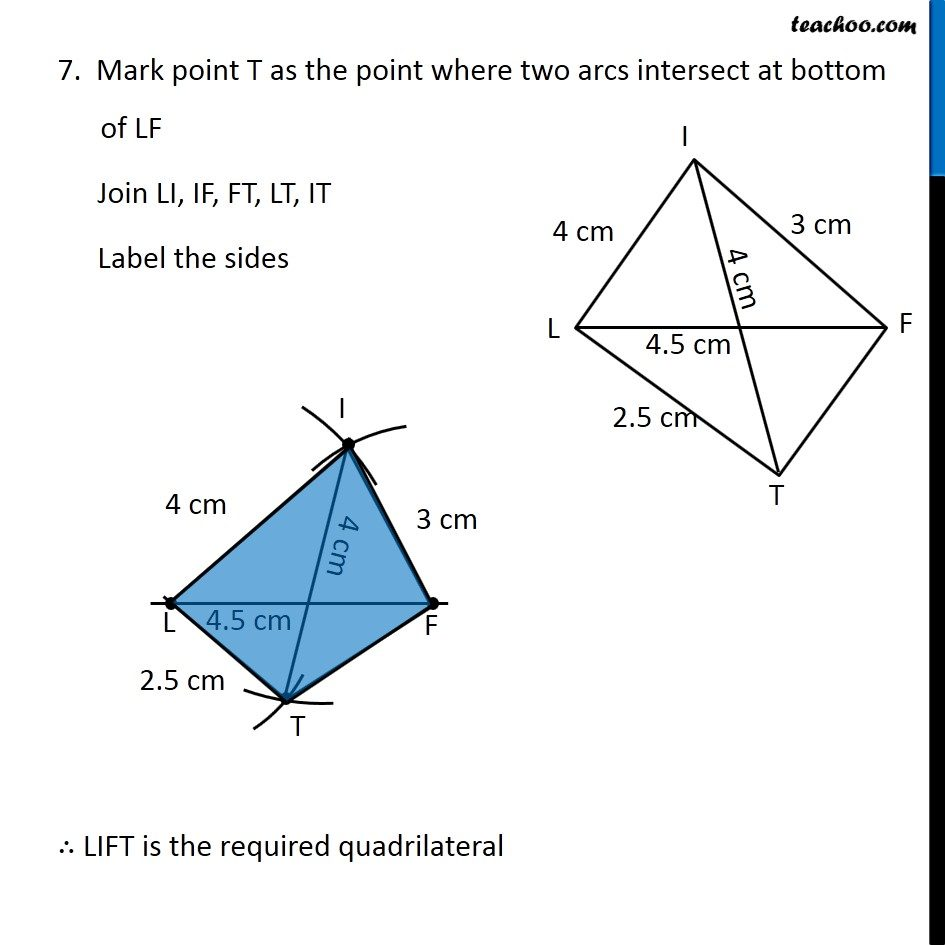1. Chapter 4 Class 8 Practical Geometry
2. Concept wise
3. Constructing Quadrilateral - 2 Diagnols and 3 sides given

Transcript

Ex 4.2, 1 (i) Construct the following quadrilaterals. Quadrilateral LIFT LI = 4 cm IF = 3 cm TL = 2.5 cm LF = 4.5 cm IT = 4 cm First we draw a rough sketch Let’s construct it Steps of construction 1. Draw diagonal LF of length 4.5 cm Steps of construction 1. Draw diagonal LF of length 4.5 cm 3. Taking 3 cm as radius, and F as center, draw an arc on top of LF 4. Mark point I as the point where two arcs intersect at top of LF 5. Now, we will mark point T Taking 2.5 cm as radius, and L as center, draw an arc on bottom of LF 6. Taking 4 cm as radius, and I as center, draw an arc on bottom of LF 7. Mark point T as the point where two arcs intersect at bottom of LF Join LI, IF, FT, LT, IT Label the sides ∴ LIFT is the required quadrilateral

Constructing Quadrilateral - 2 Diagnols and 3 sides given